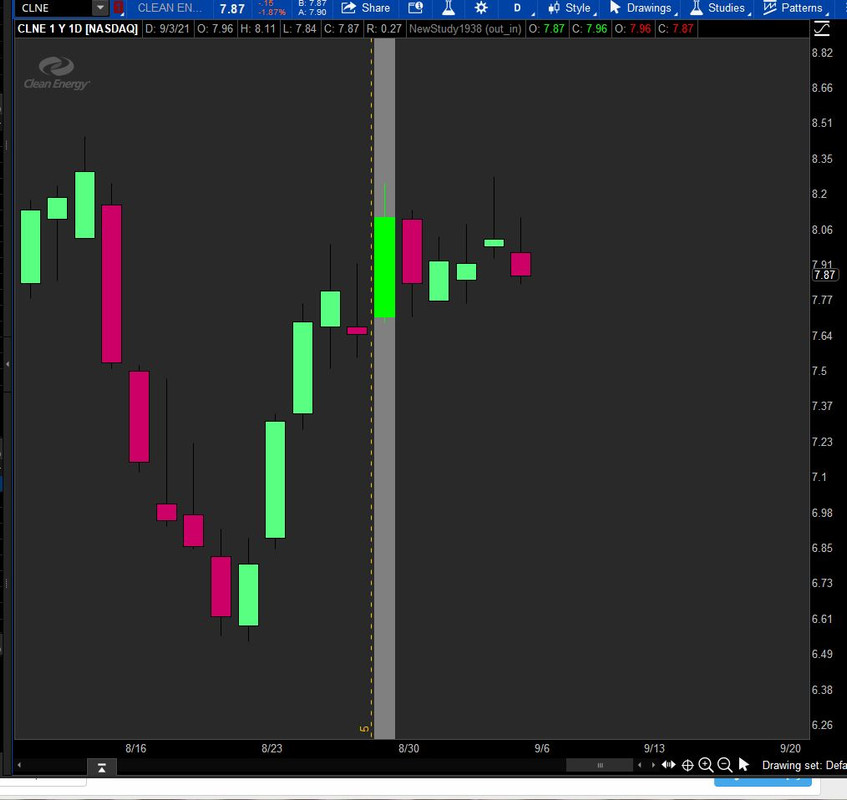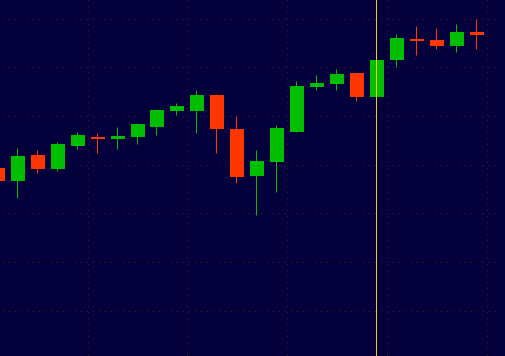# vertical line at the middles of the bar

#### michaelhasip

##### New member
I added a vertical line to indicate how far to the newest bar with below code:
Code:
``````def last_bar = HighestAll(if !IsNaN(close) then BarNumber() else Double.NEGATIVE_INFINITY);

AddVerticalLine((last_bar - BarNumber() == 5) , 5, Color.ORANGE, Curve.SHORT_DASH);``````
However, the vertical line appear at the left hand side of the bar.
Is there any chance that I could move the line to the middle of the bar? Using same code for 1min/5min/day/week chart

Solution
Nice work ...
I have the slimier scripts ... just wondering is it possible to make the vertical line thicker ? I tried but did not work ....
Here is one workaround the addverticalline() lack of functionality using the above code and adding clouds and the unsupported addchart() that Joshua mentioned.Ruby:
``````def last_bar = HighestAll(if !IsNaN(close) then BarNumber() else Double.NEGATIVE_INFINITY);

AddVerticalLine((last_bar - BarNumber() == 5) , 5, Color.ORANGE, Curve.SHORT_DASH);

#Workaround Cloud between vertical lines using addchart()

def inbar  = last_bar - BarNumber() == 6;
def outbar = last_bar - BarNumber() == 5;

def h = Double.POSITIVE_INFINITY;
def l = Double.NEGATIVE_INFINITY;
def xoutbar  = if outbar...``````
Unfortunately, that's just how it works. There is an alternative way to do it, but it involves an undocumented unsupported function which crashes the client if used incorrectly. Perhaps after the market closes I'll give it a go and let you know what I come up with.

•michaelhasip
Nice work ...
I have the slimier scripts ... just wondering is it possible to make the vertical line thicker ? I tried but did not work ....

Nice work ...
I have the slimier scripts ... just wondering is it possible to make the vertical line thicker ? I tried but did not work ....
Here is one workaround the addverticalline() lack of functionality using the above code and adding clouds and the unsupported addchart() that Joshua mentioned.Ruby:
``````def last_bar = HighestAll(if !IsNaN(close) then BarNumber() else Double.NEGATIVE_INFINITY);

AddVerticalLine((last_bar - BarNumber() == 5) , 5, Color.ORANGE, Curve.SHORT_DASH);

#Workaround Cloud between vertical lines using addchart()

def inbar  = last_bar - BarNumber() == 6;
def outbar = last_bar - BarNumber() == 5;

def h = Double.POSITIVE_INFINITY;
def l = Double.NEGATIVE_INFINITY;
def xoutbar  = if outbar then 1 else 0;
def xinbar   = if inbar  then 1 else 0;
def xboth    = if xoutbar == 1
then 1 else
if xboth == 1 and xinbar == 1
then 1 else 0;

input cloud = {default out_in, NONE};

def xH =     if cloud == cloud.NONE
then Double.NaN else
if cloud == cloud.out_in        and xoutbar  == 1
then h else Double.NaN;
def xL =     if cloud == cloud.NONE
then Double.NaN else
if cloud == cloud.out_in        and xoutbar == 1
then l else Double.NaN;

AddChart(high = xH, low = xL, open = xH, close = xL, type = ChartType.CANDLE, growColor = Color.GRAY);

##########################
#Display candles hidden by above workaround
def rh =     if cloud == cloud.NONE
then Double.NaN else
if cloud == cloud.out_in        and xoutbar == 1
then high else Double.NaN;

def rl =     if cloud == cloud.NONE
then Double.NaN else
if cloud == cloud.out_in        and xoutbar == 1
then low else Double.NaN;
def rhg = if close >= open then rh else Double.NaN;
AddChart(high = rhg, low = rl, open = close, close = open, growColor = Color.GREEN, type = ChartType.CANDLE);

def rhr = if close < open then rh else Double.NaN;
AddChart(high = rhr, low = rl, open = open, close = close, growColor = Color.RED, type = ChartType.CANDLE);``````

•••MerryDay, Topas and michaelhasip
Here is one workaround the addverticalline() lack of functionality using the above code and adding clouds and the unsupported addchart() that Joshua mentioned.
Brilliant workaround idea .... thank you .... i have been trying, could not figure out a way for limited functionality .... job done ... thanks

•SleepyZ
Here is another option as well.Ruby:
``````def Last_bar = HighestAll(
if !IsNaN(close)
then BarNumber()
else Double.NEGATIVE_INFINITY
);
def Target_bar = last_bar - BarNumber() == 5;
def DPI = if Target_bar then Double.POSITIVE_INFINITY else Double.NAN;
def DNI = if Target_bar then Double.NEGATIVE_INFINITY else Double.NAN;

Again, take caution with AddChart(), especially the chart type parameter.

Last edited:
•SleepyZ

### Not the exact question you're looking for?

87k+ Posts
296 Online## The Market Trading Game Changer

Join 2,500+ subscribers inside the useThinkScript VIP Membership Club
• Exclusive indicators
• Proven strategies & setups
• Private Discord community
• Exclusive members-only content
• 1 full year of unlimited support

What is useThinkScript?

useThinkScript is the #1 community of stock market investors using indicators and other tools to power their trading strategies. Traders of all skill levels use our forums to learn about scripting and indicators, help each other, and discover new ways to gain an edge in the markets.

How do I get started?

We get it. Our forum can be intimidating, if not overwhelming. With thousands of topics, tens of thousands of posts, our community has created an incredibly deep knowledge base for stock traders. No one can ever exhaust every resource provided on our site.

If you are new, or just looking for guidance, here are some helpful links to get you started.

What are the benefits of VIP Membership?